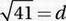# SAT Math Multiple Choice Question 635: Answer and Explanation

### Test Information

Question: 635

5.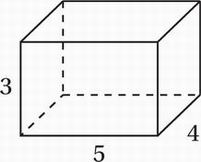The figure above shows a rectangular box. What is the longest length of a diagonal of one of the faces of this box?

• A.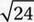• B.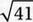• C.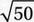• D.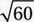Explanation:

B

Special Topics (three dimensional geometry) MEDIUM

Notice that the question asks us for the longest length of a diagonal on one of the faces of the box, and that there are three different rectangles as faces: a 3 × 4 rectangle, a 3 × 5 rectangle, and a 4 × 5 rectangle. Clearly the one with the two greatest dimensions will have the longest diagonal, which we can find using the Pythagorean Theorem.

42 + 52 = d2

Simplify:

16 + 25 = d2

Simplify:

41 = d2

Take the square root: